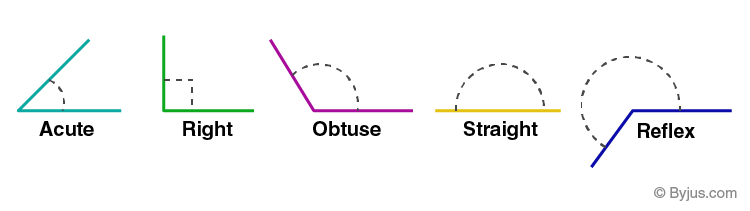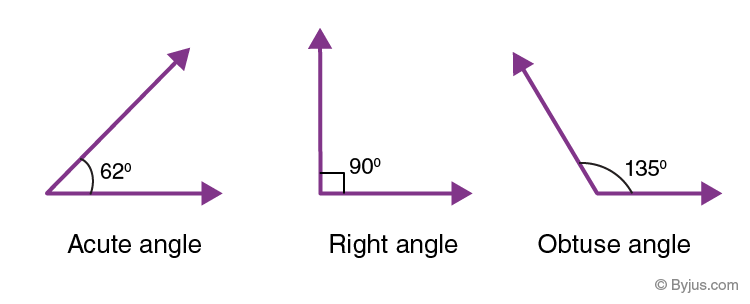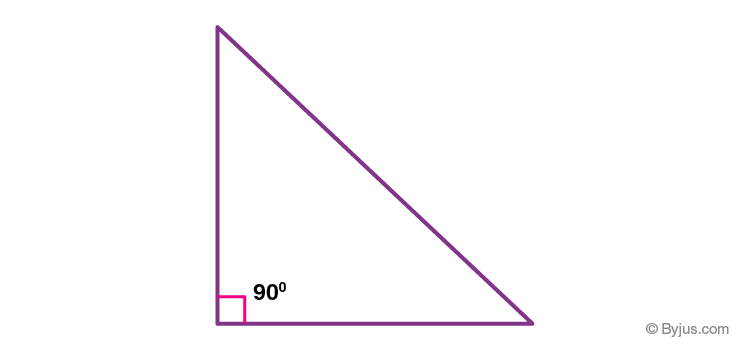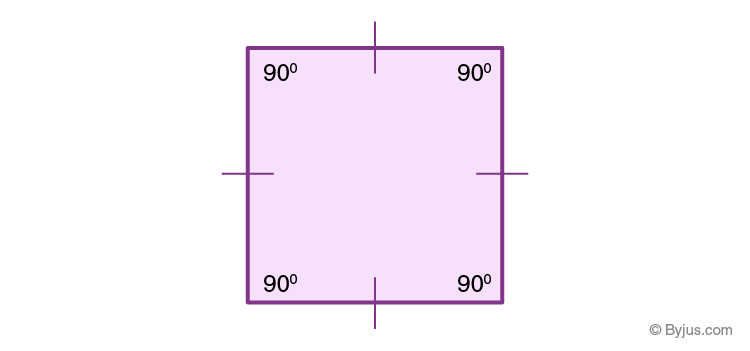# Right Angle

In geometry, the figure formed by two rays, called the sides of the angle, sharing a common endpoint, called the vertex of the angle is known as an angle. Angles created by two rays lie in the plane that contains the rays. The intersection of two planes also forms angles. The angle is also used to designate the measure of an angle or a rotation. Based on this rotation, various types of angles are defined. In this article, you will learn one of these angles, along with examples in detail.## Right Angle Definition

If the measure of angle between two rays is exactly equal to 90 degrees, then the angle is called a right angle. Angles which measure less than 90° such as 87°, 56°, 77°, 42°, etc., are acute angles and the angles which measure more than 90° such as 91°, 98°, 102°, 150°, 167°, etc., are called obtuse angles.

The measure of right angle can be written in terms of degrees as 90° and in terms of radians as π/2 (= 1.5708) radians.

## Right Angle Image

Below figure shows the shape of a right angle formed by two rays.## Right Angle Triangle

A right-angled triangle is the one which has three sides, “base” “hypotenuse” and “perpendicular” with the angle between the base and the perpendicular is 90°. A right-angled triangle is one of the basic shapes in geometry and it forms the foundation for trigonometry.In the right triangle, the hypotenuse is the longest side and is opposite the right angle of the triangle.

### Right Angle Triangle Formula

The formula used to determine whether the given triangle is the right triangle or not is Pythagoras theorem. The theorem states that the square of hypotenuse is equal to the sum of the squares of the other two sides.

(Hypotenuse)2 = (Base)2 + (Perpendicular)2

### Right Angle Triangle Properties

Some of the important properties of a right triangle are listed below.

• A right triangle has one angle exactly equal to 90 degrees
• The angles other than right angle must be acute angles, i.e. less than 90 degrees
• The side opposite to vertex of 90 degrees is called the hypotenuse of the right triangle and is the longest side of the triangle
• The other two sides adjacent to the right angle are called base and perpendicular.
• The circumcircle of the right triangle passes through all three vertices, and the radius of this circle is equal to half of the length of the hypotenuse.

### Right Angle Triangle Area

The area of a triangle is the area enclosed by three sides of the triangle in a plane. The formula to find the area of a right triangle is given by:

Area (A) = = ½ × Base × Height

Here,

Height = Perpendicular

### Right Angle Isosceles Triangle

When the two sides other than hypotenuse, i.e. base and perpendicular are congruent in a right triangle, then it is called a right angle isosceles triangle or simply isosceles right triangle. In this type of triangle, the angles made by the base and perpendicular with the hypotenuse are congruent, i.e. both measure 45 degrees each.

## Right Angle Example

We may observe right angles in many objects such as edges of a book meeting at right angles at the vertices, sides of a rectangular table or boards in classrooms forming right angles at the corners. Below figure shows the rectangular board that has right angles at its corners.We know that all the interior angles of a square are right angles, i.e. equal to 90 degrees as shown in the figure.Also, the angle formed by the x-axis and y-axis in the coordinate plane at the center (intersection of axes) are right angles.

## Frequently Asked Questions on Right Angle

### How do you define a right angle?

A right angle is defined as the angle made by two rays at a vertex exactly equal to 90 degrees. That means, both the rays are perpendicular to each other.

### What is an example of a right angle?

There are many real life examples which contain right angles such as corners of note books, tables, boards in classrooms, doors and windows of a house, which have their corners in the shape of a right angle, and so on.

### Why is it called a right angle?

A right-angle is so called a right angle since this angle forms when two rays (lines or line segments) intersect each other at 90 degrees or when they are perpendicular to each other.

### What are the 3 angles of a right triangle?

In a right triangle, the three angles include one right angle and two acute angles since the sum of all the interior angles of any triangle must be 180 degrees. Hence, a right triangle has two acute angles other than the right angle.

### What is the formula of the right angle triangle?

If a, b, c are the sides of a triangle such that a being the longest side and b, c are the perpendicular sides, then a^2 + b^2 = c^2.

This is called the formula of a right angled triangle as it helps in determining whether the given three sides belong to the right triangle or not.

Test your Knowledge on Right Angle